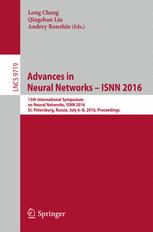• A
• A
• A
• ABC
• ABC
• ABC
• ąÉ
• ąÉ
• ąÉ
• ąÉ
• ąÉ
Regular version of the site

## Pattern Classification with the Probabilistic Neural Networks Based on Orthogonal Series Kernel

P. 505-512.

Probabilistic neural network (PNN) is the well-known instance-based learning algorithm, which is widely used in various pattern classification and regression tasks, if rather small number of instances for each class is available. The known disadvantage of this network is its insufficient classification computational complexity. The common way to overcome this drawback is the reduction techniques with selection of the most typical instances. Such approach causes the shifting of the estimates of the class probability distribution, and, in turn, the decrease of the classification accuracy. In this paper we examine another possible solution by replacing the Gaussian window and the Parzen kernel to the orthogonal series Fejér kernel and using the naïve assumption about independence of features. It is shown, that our approach makes it possible to achieve much better runtime complexity in comparison with either original PNN or its modification with the preliminary clustering of the training set.

### In bookąó. 9719: Lecture Notes in Computer Science. Switzerland: Springer, 2016.